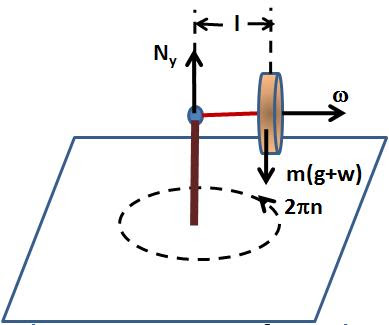## Friday, March 13, 2009

### Irodov Problem 1.284Since the entire system is accelerating upwards at a rate w, from the famous Einstein's principle of equivalence w.r.t to an observer in the accelerating frame it will be as if the acceleration due to gravity is g+w instead of g.

Let the angular velocity vector due to the spinning of the disc be. As the gyroscope undergoes precession, the entire disc moves in a circle at a rate. Hence, we can write,As seen from the bearing there is only one torque acting on the disc, that due to the force of gravity + acceleration given by m(g+w)l acting at a distance of l. Hence we have,#### 1 comment:Anonymous said...

nice problem